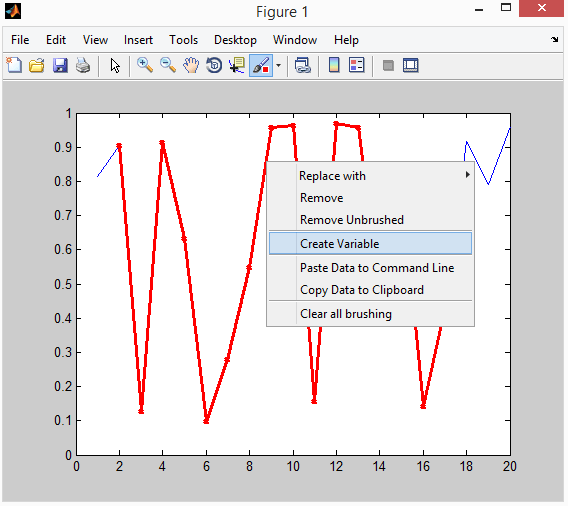There are many methods to obtain data from Matlab plots. Here I am going to show you a couple of them.

## Method 1: Select data with “Brush/Select Data” Tool in GUI

After selecting the “Brush/Select Data” tool, hold down the left button and drag through the data you want to select. Then right click the mouse to select “Create Variable” and create variable you wants to name it.It is very convenient and intuitive to do this way. The drawback of this method is that you would need a steady hand with mouse. If you are a script person, you might prefer writing a function to do it.

## Method 2: Extract data with Matlab script

If you are using R2014a or older version, you can use some script like this (courtesy to this post from Mathworks):

If you are using R2014b or newer version, the access to the graphical object become much easier. You could use this:

or simply something like this:

How to Obtain Data From Matlab Plot?
Tagged on:

### 2 thoughts on “How to Obtain Data From Matlab Plot?”

•May 26, 2017 at 2:59 pm

I am using MAtlab 2017a
I used the following code:

global xdata
global ydata
z=sin(1:100);
plot(z)
brush on
h = brush

axesObjs = h.Children(1) ;
dataObjs = axesObjs.Children(1) ;
xdata = dataObjs.XData ;
ydata = dataObjs.YData ;
zdata = dataObjs.ZData ;

but I get the error:
No appropriate method, property, or field ‘Children’ for class ‘matlab.graphics.interaction.internal.brush’.
Infact while trying it first I used the following, but xdata and ydata stored all the variables plotted already , I dont understand why

global xdata
global ydata
z=sin(1:100);
plot(z)
h = gcf ;

axesObjs = h.Children(1) ;
dataObjs = axesObjs.Children(1) ;
xdata = dataObjs.XData ;
ydata = dataObjs.YData ;

•May 26, 2017 at 3:10 pm